Reach Us+44-1522-440391
Simulating Plant Metabolic Pathways with Enzyme-Kinetic Models Using a New Approach to Homotopy Perturbation Method | OMICS International
Journal of Material Sciences & Engineering
All submissions of the EM system will be redirected to Online Manuscript Submission System. Authors are requested to submit articles directly to Online Manuscript Submission System of respective journal.

# Simulating Plant Metabolic Pathways with Enzyme-Kinetic Models Using a New Approach to Homotopy Perturbation Method

Rajendran L* and Nagarajan D

Department of Mathematics, The Madura College, Madurai, India

*Corresponding Author:
Rajendran L
Department of Mathematics
The Madura College, Madurai, India
Tel: 0452-2673354
E-mail: [email protected]

Received Date: April 16, 2015; Accepted Date: April 28, 2015; Published Date: May 08, 2015

Citation: Rajendran L, Nagarajan D (2015) Simulating Plant Metabolic Pathways with Enzyme-Kinetic Models Using a New Approach to Homotopy Perturbation Method. J Material Sci Eng 4:167. doi: 10.4172/2169-0022.1000167

Copyright: © 2015 Rajendran L, et al. This is an open-access article distributed under the terms of the Creative Commons Attribution License, which permits unrestricted use, distribution, and reproduction in any medium, provided the original author and source are credited.

Visit for more related articles at Journal of Material Sciences & Engineering

#### Abstract

The mathematical models of plant metabolic pathways are discussed. Kinetic modeling is the most detailed mathematical description of a metabolic network and constitutes an important branch in the growing fields of systems biology. This model is based on non-stationary diffusion equations containing a nonlinear term related to the Michaelis-Menten kinetics. An analytical expression of metabolic concentration was obtained for all values of parameters using new approach to Homotopy perturbation method. Our analytical results were compared with simulation results. Satisfactory agreement with simulation data is noted.

#### Keywords

Nonlinear equations; New homotopy perturbation method; Mathematical modeling; Michaelis-menten kinetics

#### Introduction

A Mathematical model is a description of a system using mathematical concepts and language. The process of developing a mathematical model is termed mathematical modeling. Mathematical models are used not only in the natural sciences, but also in the social science (such as economics, psychology, sociology, and political science). Mathematical models can take many forms, including but not limited to dynamical systems, statistical models, differential equations, or game theoretic models. Mathematical modeling of metabolism is usually closely associated with changes in compound concentrations that are described in terms of rates of biochemical reactions .

Various aspects of plant physiology have been analyzed extensively with kinetic models, and the field has a history going back for more than two decades. Detailed surveys of these models, the pathways they are addressing, and the techniques used can be found in [2,3]. A recent review was devoted to a quantitative comparison and ranking of all the kinetic models that have been published on the Calvin–Benson cycle . This information will not be repeated here; instead, the purpose of this section is 2-fold. First, a summary of recent plant kinetic models that have been published since the last comprehensive review by is presented .

Kinetic modeling is the most detailed and complex mathematical description of a metabolic network and constitutes an important branch in the growing fields of systems biology. Kinetics modelling is to express the stoichiometries and regulatory interactions in quantitative terms. The dynamics of metabolic networks are predominated by the activity of enzymes – proteins that have evolved to catalyze specific biochemical transformations. The activity and specificity of all enzymes determines the specific paths in which metabolites are broken down and utilized within a cell or compartment. Note that enzymes do not affect the position of equilibrium between substrates and products .

A detailed kinetic description of enzyme catalyzed reactions is paramount to kinetic modeling of metabolic networks-and one of the most challenging steps in the construction of large-scale models of metabolism Elaborate descriptions of the fundamentals of enzyme kinetics are found in a variety of monographs, most notably the book of among many other works on the subject .

A metabolic network can be translated in mathematical terms by relatively easy means: the concentration of a metabolite is described by a variable si. The rate of each enzymatic step can be described by enzyme kinetic rate laws, such as the Michaelis-Menten equation, as a function depending on metabolite concentration and parameters such as the maximal velocity of a reaction, or binding constants. A metabolic network that consists of m metabolic reactants (metabolites) interacting via a set of r-biochemical reactions or interconversions. Mathematical modeling of metabolism is usually closely associated with changes in compound concentrations that are described in terms of rates of biochemical reactions. More details on different methods for metabolic modelling are given in the recent comprehensive overview of computational models of metabolism . As a result, in this communication we have arrived at an analytical expression corresponding to the concentration of substrate and product using Homotopy perturbation methods for all values of reaction/diffusion parameters.

#### Mathematical Formulation of Problems and Analysis

During an enzyme-kinetic models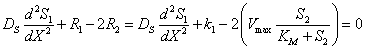(1)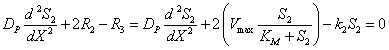(2)

Where S is imported into the modeled system by R1, converted to P by R2, and finally taken out of the system by R3 where Ki are parameters indicating the velocities of the reactions, S and P are the concentrations of the two metabolites. R1 Carries a constant flux, while R2 and R3 follow mass action kinetics; In case of R2 the product P is acting as an activator of the reaction. At this point it is important to account for reversibility as well as inhibition or activation of an enzyme, since omitting these effects is a common cause of unrealistic behavior .

A general scheme (Figure 1) that represents the change in metabolite concentration is shown below: The differential equations for the metabolites can be established from Eq. (1) and Eq. (2) respectively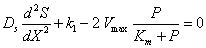(3)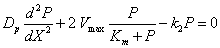(4)

where S and P are the concentrations of the two metabolites, Ds and Dp are the diffusion coefficients, Vmax are the maximal velocity of the enzymatic reaction, Km are the Michaelis- menten constant, K1, K2 are parameters indicating the velocities of the reactions. In the above equations the initial and boundary conditions are given by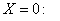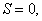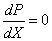(5)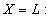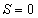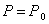(6)

We introduce the following set of dimensionless variables: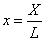,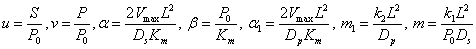(7)

The above non-linear differential equations are expressed in the following dimensionless form: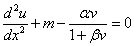(8)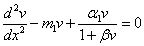(9)

Where α, α1 and β are the saturation parameters, x is the dimensionless distance, m1 and m are diffusion parameters, u and v are the dimensionless concentration. The boundary conditions in nondimensional form for the studied cases are: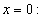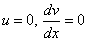(10)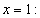u = 0, v = 1(11)

#### Analytical Expression of the Concentration Using the New Homotopyperturbation Method

Recently, many authors have applied the Homotopy perturbation method to various problems and demonstrated the efficiency of the Homotopy perturbation method for handling non-linear structures and solving various physics and engineering problems [9-12]. This method is a combination of Homotopy in topology and classic perturbation techniques. Ji-Huan He used the HPM to solve the Lighthill equation, the duffing equation and Blasius equation [13-15]. The idea has been used to solve nonlinear boundary value problems, integral equations and many other problems [16,17]. The HPM is unique in its applicability, accuracy and efficiency. The HPM uses the imbedding parameter P as a small parameter and only a few iterations are needed to search for an asymptotic solution. Using this method (Appendix A), we can obtain the following solution to Eqs. 8 and 9 (Appendix B).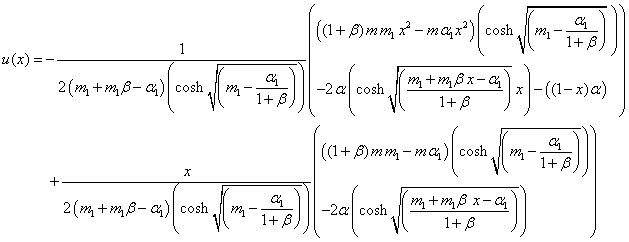(12)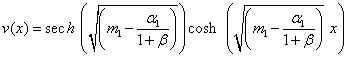(13)

Eq. (12) and Eq. (13) represent the new simple analytical expression of the concentrations for all values of parameters m, α, α1, β.

#### Discussion

Eqs. (3) and (4) represents the approximation analytical expression of concentrations of substrate S and product P. The non linear Eqs. (8)-(9) are also solved by numerical methods. Our analytical results for the numerical concentration of substrate is compared with simulation results in Figure 2. (a)-(c) for various values parameter m, α, α1, β. Also the value of concentration of substrate u(x) is equal to zero when x=0 and 1. From the Figure 2a, it is observed that the substrate concentration of u(x) is slowly increases when m increases for small value of other parameters and then it reaches the maximum values at x=0.5. Similarly, in Figure 2b, the value of concentration of substrate u(x) is slowly decreases when β decrease for large value of other parameters. Also value of concentration substrate u(x) increases when α decreases for large value of other parameters (Figure 2c).

Figure 2: Normalized concentration profiles of substrate u(x) from Eq. (12), for different values ofm, α , α1 and β when (a) α1 = .01, α = .01, β = .01, m1 = .01 (b) 3 α1 = , α = 3 , 3 m1 = , m = 3 (c) α1 = 3, β = 3, β = 3, m = 3 .Solid lines represent the analytical solution obtained in this work; dotted lines represent the numerical solution.

Figure 3(a)-(c) represents the comparison of analytical expression of concentration of product with simulation results from the Figure 3a it is inferred that the concentration of product v(x) is slowly increases when small values of m1. In Figure 3b the product concentration v(x) is increases when β decreases for large value of α1 and m1. Also value of concentration of product v(x) increases when α1 increases for different value of saturation parameter β and diffusion parameter m1 (Figure 3c).

Figure 3: Normalized concentration profiles of product v(x) from Eq. (13), for different values of m1 , α1 and β when (a) α1 = .0001,β = .01 (b) α1 = 65, m1 = 65 (c)β = .01, m1 = 65 . Solid lines represent the analytical solution obtained in this work; dotted lines represent the numerical solution.

#### Conclusion

The time independent, non- linear reaction/diffusion equation has been formulated and solved analytically. An approximate analytical expression for the concentration of substrate and product are obtained by using the Homotopy perturbation method. The primary result of this work is simple approximate calculation of concentration for all possible values of parameters. This method can be easily extended to find the solution of all other non-linear reaction diffusion equations in metabolic modeling for various complex boundary conditions.

#### References

Select your language of interest to view the total content in your interested language

### Article Usage

• Total views: 12034
• [From(publication date):
June-2015 - Aug 19, 2019]
• Breakdown by view type
• HTML page views : 8234
• PDF downloads : 3800

## Post your commentCan't read the image? click here to refresh
###### Peer Reviewed Journals

Make the best use of Scientific Research and information from our 700 + peer reviewed, Open Access Journals

International Conferences 2019-20

Meet Inspiring Speakers and Experts at our 3000+ Global Annual Meetings

Top